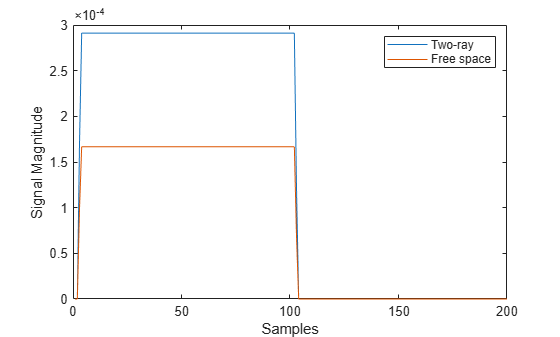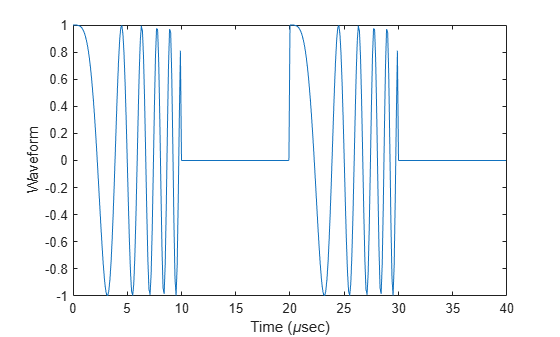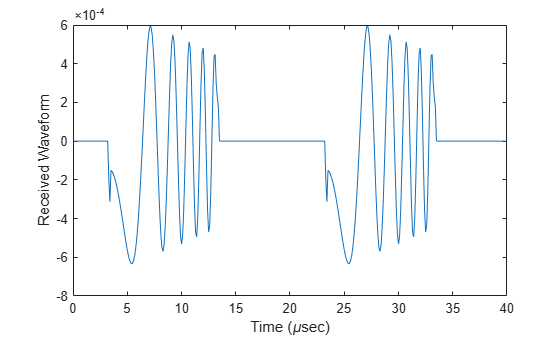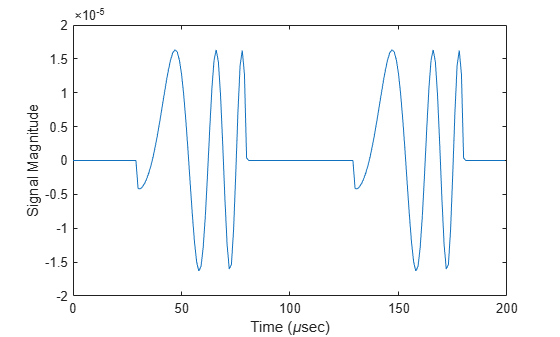# step

System object: twoRayChannel

Propagate signal from point to point using two-ray channel model

## Syntax

prop_sig = step(channel,sig,origin_pos,dest_pos,origin_vel,dest_vel) 

## Description

Note

Starting in R2016b, instead of using the step method to perform the operation defined by the System object™, you can call the object with arguments, as if it were a function. For example, y = step(obj,x) and y = obj(x) perform equivalent operations.

prop_sig = step(channel,sig,origin_pos,dest_pos,origin_vel,dest_vel) returns the resulting signal, prop_sig, when a narrowband signal, sig, propagates through a two-ray channel from the origin_pos position to the dest_pos position. Either the origin_pos or dest_pos arguments can have multiple points but you cannot specify both as having multiple points. The velocity of the signal origin is specified in origin_vel and the velocity of the signal destination is specified in dest_vel. The dimensions of origin_vel and dest_vel must agree with the dimensions of origin_pos and dest_pos, respectively.

Electromagnetic fields propagated through a two-ray channel can be polarized or nonpolarized. For, nonpolarized fields, such as an acoustic field, the propagating signal field, sig, is a vector or matrix. When the fields are polarized, sig is an array of structures. Every structure element represents an electric field vector in Cartesian form.

In the two-ray environment, there are two signal paths connecting every signal origin and destination pair. For N signal origins (or N signal destinations), there are 2N number of paths. The signals for each origin-destination pair do not have to be related. The signals along the two paths for any single source-destination pair can also differ due to phase or amplitude differences.

You can keep the two signals at the destination separate or combined — controlled by the CombinedRaysOutput property. Combined means that the signals at the source propagate separately along the two paths but are coherently summed at the destination into a single quantity. To use the separate option, set CombinedRaysOutput to false. To use the combined option, set CombinedRaysOutput to true. This option is convenient when the difference between the sensor or array gains in the directions of the two paths is not significant and need not be taken into account.

Note

The object performs an initialization the first time the object is executed. This initialization locks nontunable properties and input specifications, such as dimensions, complexity, and data type of the input data. If you change a nontunable property or an input specification, the System object issues an error. To change nontunable properties or inputs, you must first call the release method to unlock the object.

## Input Arguments

expand all

Two-ray channel, specified as a System object.

Example: twoRayChannel

• Narrowband nonpolarized scalar signal, specified as an

• M-by-N complex-valued matrix. Each column contains a common signal propagated along both the line-of-sight path and the reflected path. You can use this form when both path signals are the same.

• M-by-2N complex-valued matrix. Each adjacent pair of columns represents a different channel. Within each pair, the first column represents the signal propagated along the line-of-sight path and the second column represents the signal propagated along the reflected path.

• Narrowband polarized signal, specified as a

• 1-by-N struct array containing complex-valued fields. Each struct contains a common polarized signal propagated along both the line-of-sight path and the reflected path. Each structure element contains an M-by-1 column vector of electromagnetic field components (sig.X,sig.Y,sig.Z). You can use this form when both path signals are the same.

• 1-by-2N struct array containing complex-valued fields. Each adjacent pair of array columns represents a different channel. Within each pair, the first column represents the signal along the line-of-sight path and the second column represents the signal along the reflected path. Each structure element contains an M-by-1 column vector of electromagnetic field components (sig.X,sig.Y,sig.Z).

For nonpolarized fields, the quantity M is the number of samples of the signal and N is the number of two-ray channels. Each channel corresponds to a source-destination pair.

The size of the first dimension of the input matrix can vary to simulate a changing signal length. A size change can occur, for example, in the case of a pulse waveform with variable pulse repetition frequency.

For polarized fields, the struct element contains three M-by-1 complex-valued column vectors, sig.X, sig.Y, and sig.Z. These vectors represent the x, y, and z Cartesian components of the polarized signal.

The size of the first dimension of the matrix fields within the struct can vary to simulate a changing signal length such as a pulse waveform with variable pulse repetition frequency.

Example: [1,1;j,1;0.5,0]

Data Types: double
Complex Number Support: Yes

Origin of the signal or signals, specified as a 3-by-1 real-valued column vector or 3-by-N real-valued matrix. The quantity N is the number of two-ray channels. If origin_pos is a column vector, it takes the form [x;y;z]. If origin_pos is a matrix, each column specifies a different signal origin and has the form [x;y;z]. Position units are meters.

origin_pos and dest_pos cannot both be specified as matrices — at least one must be a 3-by-1 column vector.

Example: [1000;100;500]

Data Types: double

Destination position of the signal or signals, specified as a 3-by-1 real-valued column vector or 3-by-N real-valued matrix. The quantity N is the number of two-ray channels propagating from or to N signal origins. If dest_pos is a 3-by-1 column vector, it takes the form [x;y;z]. If dest_pos is a matrix, each column specifies a different signal destination and takes the form [x;y;z] Position units are in meters.

You cannot specify origin_pos and dest_pos as matrices. At least one must be a 3-by-1 column vector.

Example: [0;0;0]

Data Types: double

Velocity of signal origin, specified as a 3-by-1 real-valued column vector or 3-by-N real-valued matrix. The dimensions of origin_vel must match the dimensions of origin_pos. If origin_vel is a column vector, it takes the form [Vx;Vy;Vz]. If origin_vel is a 3-by-N matrix, each column specifies a different origin velocity and has the form [Vx;Vy;Vz]. Velocity units are in meters per second.

Example: [10;0;5]

Data Types: double

Velocity of signal destinations, specified as a 3-by-1 real-valued column vector or 3–by-N real-valued matrix. The dimensions of dest_vel must match the dimensions of dest_pos. If dest_vel is a column vector, it takes the form [Vx;Vy;Vz]. If dest_vel is a 3-by-N matrix, each column specifies a different destination velocity and has the form [Vx;Vy;Vz] Velocity units are in meters per second.

Example: [0;0;0]

Data Types: double

## Output Arguments

expand all

• Narrowband nonpolarized scalar signal, returned as an:

• M-by-N complex-valued matrix. To return this format, set the CombinedRaysOutput property to true. Each matrix column contains the coherently combined signals from the line-of-sight path and the reflected path.

• M-by-2N complex-valued matrix. To return this format set the CombinedRaysOutput property to false. Alternate columns of the matrix contain the signals from the line-of-sight path and the reflected path.

• Narrowband polarized scalar signal, returned as:

• 1-by-N struct array containing complex-valued fields. To return this format, set the CombinedRaysOutput property to true. Each column of the array contains the coherently combined signals from the line-of-sight path and the reflected path. Each structure element contains the electromagnetic field vector (prop_sig.X,prop_sig.Y,prop_sig.Z).

• 1-by-2N struct array containing complex-valued fields. To return this format, set the CombinedRaysOutput property to false. Alternate columns contains the signals from the line-of-sight path and the reflected path. Each structure element contains the electromagnetic field vector (prop_sig.X,prop_sig.Y,prop_sig.Z).

The output prop_sig contains signal samples arriving at the signal destination within the current input time frame. Whenever it takes longer than the current time frame for the signal to propagate from the origin to the destination, the output may not contain all contributions from the input of the current time frame. The remaining output will appear in the next call to step.

## Examples

expand all

Propagate a signal in a two-ray channel environment from a radar at (0,0,10) meters to a target at (300,200,30) meters. Assume that the radar and target are stationary and that the transmitting antenna has a cosine pattern. Compare the combined signals from the two paths with the single signal resulting from free space propagation. Set the CombinedRaysOutput to true to produce a combined propagated signal.

Create a Rectangular Waveform

Set the sample rate to 2 MHz.

fs = 2e6; waveform = phased.RectangularWaveform('SampleRate',fs); wavfrm = waveform();

Create the Transmitting Antenna and Radiator

Set up a phased.Radiator System object™ to transmit from a cosine antenna

antenna = phased.CosineAntennaElement; radiator = phased.Radiator('Sensor',antenna);

Specify Transmitter and Target Coordinates

posTx = [0;0;10]; posTgt = [300;200;30]; velTx = [0;0;0]; velTgt = [0;0;0];

Free Space Propagation

Compute the transmitting direction toward the target for the free-space model. Then, radiate the signal.

[~,angFS] = rangeangle(posTgt,posTx); wavTx = radiator(wavfrm,angFS);

Propagate the signal to the target.

fschannel = phased.FreeSpace('SampleRate',waveform.SampleRate); yfs = fschannel(wavTx,posTx,posTgt,velTx,velTgt); release(radiator);

Two-Ray Propagation

Compute the two transmit angles toward the target for line-of-sight (LOS) path and reflected paths. Compute the transmitting directions toward the target for the two rays. Then, radiate the signals.

[~,angTwoRay] = rangeangle(posTgt,posTx,'two-ray'); wavTwoRay = radiator(wavfrm,angTwoRay);

Propagate the signals to the target.

channel = twoRayChannel('SampleRate',waveform.SampleRate,... 'CombinedRaysOutput',true); y2ray = channel(wavTwoRay,posTx,posTgt,velTx,velTgt);

Plot the Propagated Signals

Plot the combined signal against the free-space signal

plot(abs([y2ray yfs])) legend('Two-ray','Free space') xlabel('Samples') ylabel('Signal Magnitude')Create a polarized electromagnetic field consisting of linear FM waveform pulses. Propagate the field from a stationary source with a crossed-dipole antenna element to a stationary receiver approximately 10 km away. The transmitting antenna is 100 meters above the ground. The receiving antenna is 150 m above the ground. The receiving antenna is also a crossed-dipole. Plot the received signal.

Assume the pulse width is $10\mu s$ and the sampling rate is 10 MHz. The bandwidth of the pulse is 1 MHz. Assume a 50% duty cycle in which the pulse width is one-half the pulse repetition interval. Create a two-pulse wave train. Assume a carrier frequency of 100 MHz.

c = physconst('LightSpeed'); fs = 10e6; pw = 10e-6; pri = 2*pw; PRF = 1/pri; fc = 100e6; bw = 1e6; lambda = c/fc;

Set Up Required System Objects

Use a GroundRelativePermittivity of 10.

waveform = phased.LinearFMWaveform('SampleRate',fs,'PulseWidth',pw,... 'PRF',PRF,'OutputFormat','Pulses','NumPulses',2,'SweepBandwidth',bw,... 'SweepDirection','Up','Envelope','Rectangular','SweepInterval',... 'Positive'); antenna = phased.CrossedDipoleAntennaElement(... 'FrequencyRange',[50,200]*1e6); radiator = phased.Radiator('Sensor',antenna,'OperatingFrequency',fc,... 'Polarization','Combined'); channel = twoRayChannel('SampleRate',fs,... 'OperatingFrequency',fc,'CombinedRaysOutput',false,... 'EnablePolarization',true,'GroundRelativePermittivity',10); collector = phased.Collector('Sensor',antenna,'OperatingFrequency',fc,... 'Polarization','Combined');

Set Up Scene Geometry

Specify transmitter and receiver positions, velocities, and orientations. Place the source and receiver about 1000 m apart horizontally and approximately 50 m apart vertically.

posTx = [0;100;100]; posRx = [1000;0;150]; velTx = [0;0;0]; velRx = [0;0;0]; laxRx = rotz(180); laxTx = rotx(1)*eye(3);

Create and Radiate Signals from Transmitter

Compute the transmission angles for the two rays traveling toward the receiver. These angles are defined with respect to the transmitter local coordinate system. The phased.Radiator System object™ uses these angles to apply separate antenna gains to the two signals.

[rng,angsTx] = rangeangle(posRx,posTx,laxTx,'two-ray'); wav = waveform();

Plot the transmitted Waveform

n = size(wav,1); plot((0:(n-1))/fs*1000000,real(wav)) xlabel('Time ({\mu}sec)') ylabel('Waveform')sig = radiator(wav,angsTx,laxTx);

Propagate signals to receiver via two-ray channel

prop_sig = channel(sig,posTx,posRx,velTx,velRx);

Compute the reception angles for the two rays arriving at the receiver. These angles are defined with respect to the receiver local coordinate system. The phased.Collector System object™ uses these angles to apply separate antenna gains to the two signals.

[~,angsRx] = rangeangle(posTx,posRx,laxRx,'two-ray');

y = collector(prop_sig,angsRx,laxRx);

plot((0:(n-1))/fs*1000000,real(y)) xlabel('Time ({\mu}sec)') ylabel('Received Waveform')Propagate a linear FM signal in a two-ray channel. The signal propagates from a transmitter located at (1000,10,10) meters in the global coordinate system to a receiver at (10000,200,30) meters. Assume that the transmitter and the receiver are stationary and that they both have cosine antenna patterns. Plot the received signal.

Set up the radar scenario. First, create the required System objects.

waveform = phased.LinearFMWaveform('SampleRate',1000000,... 'OutputFormat','Pulses','NumPulses',2); fs = waveform.SampleRate; antenna = phased.CosineAntennaElement; radiator = phased.Radiator('Sensor',antenna); collector = phased.Collector('Sensor',antenna); channel = twoRayChannel('SampleRate',fs,... 'CombinedRaysOutput',false,'GroundReflectionCoefficient',0.95);

Set up the scene geometry. Specify transmitter and receiver positions and velocities. The transmitter and receiver are stationary.

posTx = [1000;10;10]; posRx = [10000;200;30]; velTx = [0;0;0]; velRx = [0;0;0];

Specify the transmitting and receiving radar antenna orientations with respect to the global coordinates. The transmitting antenna points along the +x direction and the receiving antenna points near but not directly in the -x direction.

laxTx = eye(3); laxRx = rotx(5)*rotz(170);

Compute the transmission angles which are the angles that the two rays traveling toward the receiver leave the transmitter. The phased.Radiator System object™ uses these angles to apply separate antenna gains to the two signals. Because the antenna gains depend on path direction, you must transmit and receive the two rays separately.

[~,angTx] = rangeangle(posRx,posTx,laxTx,'two-ray');

Create and radiate signals from transmitter along the transmission directions.

wavfrm = waveform(); wavtrans = radiator(wavfrm,angTx);

Propagate signals to receiver via two-ray channel.

wavrcv = channel(wavtrans,posTx,posRx,velTx,velRx);

Collect signals at the receiver. Compute the angle at which the two rays traveling from the transmitter arrive at the receiver. The phased.Collector System object™ uses these angles to apply separate antenna gains to the two signals.

[~,angRcv] = rangeangle(posTx,posRx,laxRx,'two-ray');

Collect and combine the two received rays.

yR = collector(wavrcv,angRcv);

dt = 1/fs; n = size(yR,1); plot((0:(n-1))*dt*1000000,real(yR)) xlabel('Time ({\mu}sec)') ylabel('Signal Magnitude')Propagate a 100 Mhz linear FM signal into a two-ray channel. Assume there is signal loss caused by atmospheric gases and rain. The signal propagates from a transmitter located at (0,0,0) meters in the global coordinate system to a receiver at (10000,200,30) meters. Assume that the transmitter and the receiver are stationary and that they both have cosine antenna patterns. Plot the received signal. Set the dry air pressure to 102.5 Pa and the rain rate to 5 mm/hr.

waveform = phased.LinearFMWaveform('SampleRate',1e6,... 'OutputFormat','Pulses','NumPulses',2); antenna = phased.CosineAntennaElement; radiator = phased.Radiator('Sensor',antenna); collector = phased.Collector('Sensor',antenna); channel = twoRayChannel('SampleRate',waveform.SampleRate,... 'CombinedRaysOutput',false,'GroundReflectionCoefficient',0.95,... 'SpecifyAtmosphere',true,'Temperature',20,... 'DryAirPressure',102.5,'RainRate',5.0);

Set up the scene geometry giving. the transmitter and receiver positions and velocities. The transmitter and receiver are stationary.

posTx = [0;0;0]; posRx = [10000;200;30]; velTx = [0;0;0]; velRx = [0;0;0];

Specify the transmitting and receiving radar antenna orientations with respect to the global coordinates. The transmitting antenna points along the +x-direction and the receiving antenna points close to the –x-direction.

laxTx = eye(3); laxRx = rotx(5)*rotz(170);

Compute the transmission angles which are the angles that the two rays traveling toward the receiver leave the transmitter. The phased.Radiator System object™ uses these angles to apply separate antenna gains to the two signals. Because the antenna gains depend on path direction, you must transmit and receive the two rays separately.

[~,angTx] = rangeangle(posRx,posTx,laxTx,'two-ray');

Create and Radiate Signals from Transmitter

Radiate the signals along the transmission directions.

wavfrm = waveform(); wavtrans = radiator(wavfrm,angTx);

Propagate signals to receiver via two-ray channel.

wavrcv = channel(wavtrans,posTx,posRx,velTx,velRx);

Compute the angle at which the two rays traveling from the transmitter arrive at the receiver. The phased.Collector System object™ uses these angles to apply separate antenna gains to the two signals.

[~,angRcv] = rangeangle(posTx,posRx,laxRx,'two-ray');

Collect and combine the two received rays.

yR = collector(wavrcv,angRcv);

dt = 1/waveform.SampleRate; n = size(yR,1); plot((0:(n-1))*dt*1000000,real(yR)) xlabel('Time ({\mu}sec)') ylabel('Signal Magnitude')## References

 Proakis, J. Digital Communications. New York: McGraw-Hill, 2001.

 Skolnik, M. Introduction to Radar Systems, 3rd Ed. New York: McGraw-Hill

 Saakian, A.Radio Wave Propagation Fundamentals. Norwood, MA: Artech House, 2011.

 Balanis, C.Advanced Engineering Electromagnetics. New York: Wiley & Sons, 1989.

 Rappaport, T.Wireless Communications: Principles and Practice, 2nd Ed New York: Prentice Hall, 2002.

## Extended Capabilities

### C/C++ Code GenerationGenerate C and C++ code using MATLAB® Coder™.

Introduced in R2021a